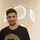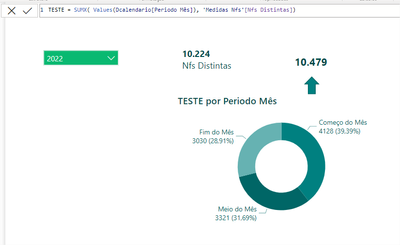cancel
Showing results for
Did you mean:Helper II

## different value in different visuals

I have a measure that has the correct total value, when I put it in a table it keeps the correct value in the total, when I break it in it changes the value, do you know what it can be?6 REPLIES 6Community Support

Hi, @Faber13 ;

I create a simple file like below:And Created visual， however For my simple example, I am not very clear, you want the output of the result, or can you create a simple example and hope to output the result display?Solved: How to upload PBI in Community - Microsoft Power BI Community

Best Regards,
Community Support Team _ Yalan Wu
If this post helps, then please consider Accept it as the solution to help the other members find it more quickly.Helper II

in my example, I need the total value breakdown to be 37,669 which is the correct value, the relationship is active for the column I'm using in the measure, I tried using "USERELATIONSHIP"Super User

The correct values is 37,669 fot the total, but not for the breakdown as you might have the same nfs in different periods so it will count one nfs per each. On the other hand, your total will do a DISTINCT over nfs before counting them, so if you previously had the same nfs, one on each perdiod, for your total it will only count as 1, thats why your total is always displaying a lower value that the sum of each period.Helper II

@PabloDeheza  that might be it, would you know how to calculate only these repeated values ​​and then leave only the last values ​​(of the repeated ones)Super User

Hi @Faber13 !
In your measure you are using DISTINCTCOUT(), which for each of the categories is counting the distinct values, the this is that for your total is its doing the same thing, so it will remove the duplicates that you may have between the categories. In other words, if one [Nota fiscal] is in both Meio do mes and Fim do mes, in your total will count as one.

If your goal is to sum the values of your categories to show that in the total then try the following:

``````SUMX( Values( Periodo Mes), [Nfs Distintas] )
``````

Let me know if that helps!Helper II

@PabloDeheza  still with the same error